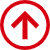# How to calculate the weight of a square steel pipe with rounded corners?

Square or rectangular steel pipes are commonly used in construction projects and are generally used for pipe installation supports, temporary site access, power projects, decorative keel, etc.

When the size of the rectangular steel pipe is large enough, we are able to disregard the effect of rounded corners on the weight, for example, rectangular duct in the duct, the weight lost by the four rounded corners is negligible for a very large section.

However, for small cross-sectional square steel pipe, rectangular steel pipe, it is necessary to consider the radius of the rounded corners R when calculating the weight. In general, the test does not take into account the weight difference in about 5% to 10%, which is related to the cost of thin material manufacturers. So how to calculate the unit weight per linear meter of square steel rectangular steel pipe with R corner?

To calculate the weight per unit length of the profile, we generally have to find out the cross-sectional area and then multiply it by the corresponding material density, and we can get its unit weight.

For the rectangular tube shown below, we can easily obtain the cross-sectional area A = H*B-(H-2t)*(B-2t) for the whole section if we do not consider the effect of R-angle.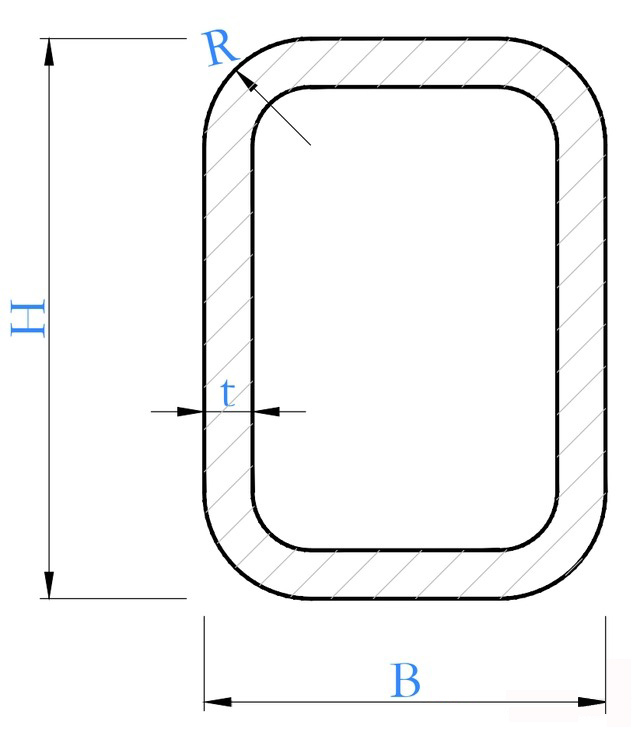Once the radius of the outer corner is known to be R, we simply add the area of the blue block and subtract the area of the red block (as shown in the figure below) to get the true area of the section. And, for most rectangular tubes, the inner and outer corners are the same circle center, so the inner corner radius r = R-t. After we figure out these points, we can easily derive the area formula.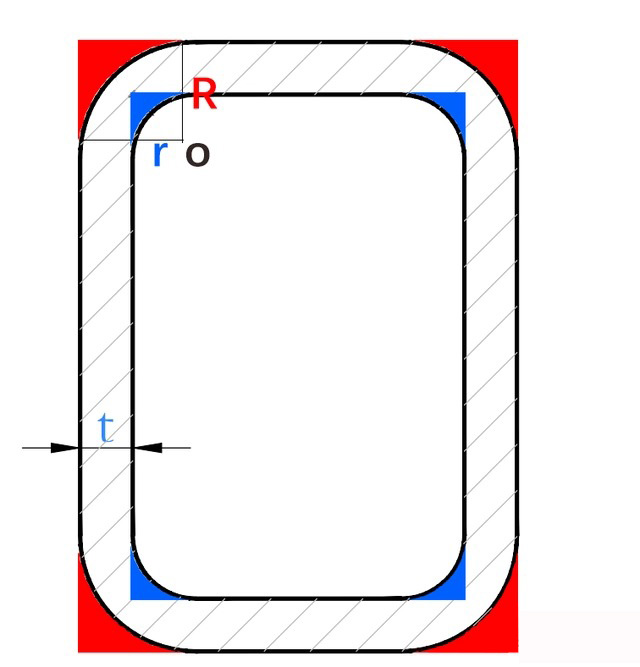In addition, in the specification "GBT 3094-2012 cold-drawn shaped steel pipe", has given a specific single weight calculation formula, its calculation principle and I said above is also consistent, you can refer to the following chart.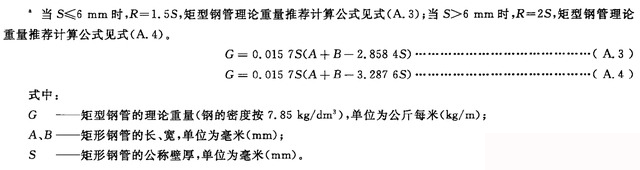Observe the formula, we can find that the value of R in the specification is determined by the wall thickness of the steel pipe, so when you do not know the specific R value for how much, you can also take the value according to the specification, that is -

### R=1.5 times the wall thickness when the wall thickness is ≤6mm. When the wall thickness >6mm, R=2 times the wall thickness

In practice, we can also calculate the unit weight of rectangular tubes with rounded corners directly using the tools at our disposal.

Open the official website of Yuantai Derun Steel Pipe Manufacturing Group, click "Contact us", find "Whatsapp" or email: sales@ytdrgg.com, after entering the module, we can directly find After entering the module, we can directly find the account manager to calculate the weight of rectangular pipe square tube.

Yuantai Delun is mainly engaged in "square steel pipe", "rectangular steel pipe" and "round steel pipe", we can consult and order with reference to our own situation. Finally, by clicking the form button at the bottom right corner, we can learn more specific parameters, including the surface area and mechanical properties of the profile, which are very detailed.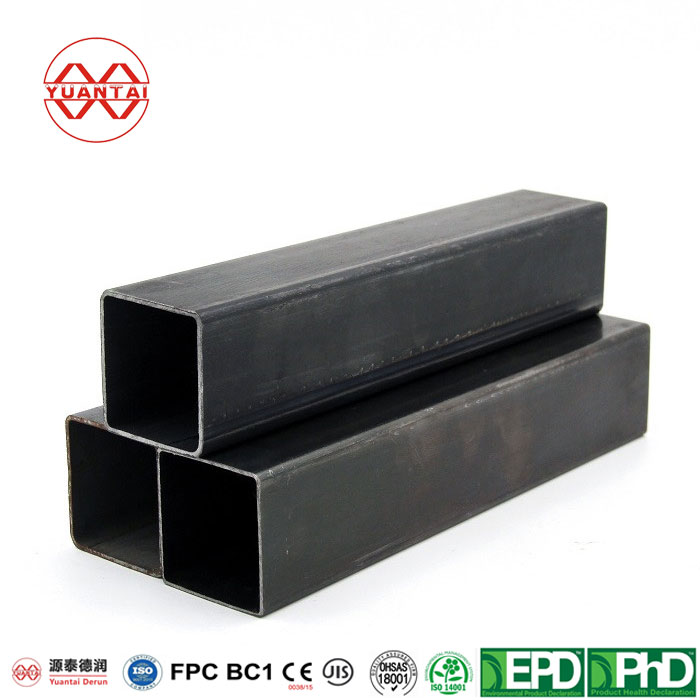Post time: Mar-13-2023
•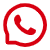#### Whatsapp

##### whatsapp
8613682051821
•